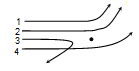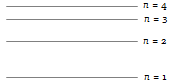Rutherford’s $\mathrm{\alpha }$-particle experiment showed that the atoms have
(a) Proton          (b) Nucleus
(c) Neutron        (d) Electrons

Concept Questions :-

Various atomic model

Difficulty Level:

Which of the following is true for number of spectral lines in going form Layman series to Pfund series
(a) Increases
(b) Decreases
(c) Unchanged
(d) May decreases or increases

Concept Questions :-

Spectral series
High Yielding Test Series + Question Bank - NEET 2020

Difficulty Level:

Radius of the first orbit of the electron in a hydrogen atom is 0.53 Å. So, the radius of the third orbit will be

(a) 2.12 Å             (b) 4.77 Å
(c) 1.06 Å             (d) 1.59 Å

Concept Questions :-

Bohr's model of atom
High Yielding Test Series + Question Bank - NEET 2020

Difficulty Level:

The first line in the Lyman series has wavelength $\mathrm{\lambda }$. The wavelength of the first line in Balmer series is

(a) $\frac{2}{9}\mathrm{\lambda }$              (b) $\frac{9}{2}\mathrm{\lambda }$
(c) $\frac{5}{27}\mathrm{\lambda }$            (d) $\frac{27}{5}\mathrm{\lambda }$

Concept Questions :-

Spectral series
High Yielding Test Series + Question Bank - NEET 2020

Difficulty Level:

In the following transitions, which one has higher frequency

(a) 3 – 2              (b) 4 – 3
(c) 4 – 2              (d) 3 – 1

Concept Questions :-

Bohr's model of atom
High Yielding Test Series + Question Bank - NEET 2020

Difficulty Level:

The diagram shows the path of four $\alpha$-particles of the same energy being scattered by the nucleus of an atom simultaneously. Which of these are/is not physically possible?(a) 3 and 4            (b) 2 and 3
(c) 1 and 4            (d) 4 only

Concept Questions :-

Various atomic model
High Yielding Test Series + Question Bank - NEET 2020

Difficulty Level:

An electron jumps from 5th orbit to 4th orbit of hydrogen atom. Taking the Rydberg constant as  ${10}^{7}$ per metre. What will be the frequency of radiation emitted

(a)               (b)
(c)               (d) None of these

Concept Questions :-

Bohr's model of atom
High Yielding Test Series + Question Bank - NEET 2020

Difficulty Level:

Four lowest energy levels of H-atom are shown in the figure. The number of possible emission lines would be(a) 3             (b) 4
(c) 5             (d) 6

Concept Questions :-

Spectral series
High Yielding Test Series + Question Bank - NEET 2020

Difficulty Level:

The order of the size of nucleus and Bohr radius of an atom respectively are
(a)                (b)
(c)                (d)

Concept Questions :-

Bohr's model of atom
High Yielding Test Series + Question Bank - NEET 2020

Difficulty Level:

The ratio of the wavelengths for 2 $\to$ 1 transition in ${\mathrm{Li}}^{++}$${\mathrm{He}}^{+}$ and H is
(a) 1 : 2 : 3            (b) 1 : 4 : 9
(c) 4 : 9 : 36           (d) 3 : 2 : 1

Concept Questions :-

Spectral series